# Subgraphs#

Example of partitioning a directed graph with nodes labeled as supported and unsupported nodes into a list of subgraphs that contain only entirely supported or entirely unsupported nodes. Adopted from lobpcg/python_examples

```import networkx as nx
import matplotlib.pyplot as plt

def graph_partitioning(G, plotting=True):
"""Partition a directed graph into a list of subgraphs that contain
only entirely supported or entirely unsupported nodes.
"""
# Categorize nodes by their node_type attribute
supported_nodes = {n for n, d in G.nodes(data="node_type") if d == "supported"}
unsupported_nodes = {n for n, d in G.nodes(data="node_type") if d == "unsupported"}

# Make a copy of the graph.
H = G.copy()
# Remove all edges connecting supported and unsupported nodes.
H.remove_edges_from(
(n, nbr, d)
if n in supported_nodes
for nbr, d in nbrs.items()
if nbr in unsupported_nodes
)
H.remove_edges_from(
(n, nbr, d)
if n in unsupported_nodes
for nbr, d in nbrs.items()
if nbr in supported_nodes
)

# Collect all removed edges for reconstruction.
G_minus_H = nx.DiGraph()

if plotting:
# Plot the stripped graph with the edges removed.
_node_colors = [c for _, c in H.nodes(data="node_color")]
_pos = nx.spring_layout(H)
plt.figure(figsize=(8, 8))
nx.draw_networkx_edges(H, _pos, alpha=0.3, edge_color="k")
nx.draw_networkx_nodes(H, _pos, node_color=_node_colors)
nx.draw_networkx_labels(H, _pos, font_size=14)
plt.axis("off")
plt.title("The stripped graph with the edges removed.")
plt.show()
# Plot the the edges removed.
_pos = nx.spring_layout(G_minus_H)
plt.figure(figsize=(8, 8))
ncl = [G.nodes[n]["node_color"] for n in G_minus_H.nodes]
nx.draw_networkx_edges(G_minus_H, _pos, alpha=0.3, edge_color="k")
nx.draw_networkx_nodes(G_minus_H, _pos, node_color=ncl)
nx.draw_networkx_labels(G_minus_H, _pos, font_size=14)
plt.axis("off")
plt.title("The removed edges.")
plt.show()

# Find the connected components in the stripped undirected graph.
# And use the sets, specifying the components, to partition
# the original directed graph into a list of directed subgraphs
# that contain only entirely supported or entirely unsupported nodes.
subgraphs = [
H.subgraph(c).copy() for c in nx.connected_components(H.to_undirected())
]

return subgraphs, G_minus_H
```

## Create an example directed graph.#

This directed graph has one input node labeled `in` and plotted in blue color and one output node labeled `out` and plotted in magenta color. The other six nodes are classified as four `supported` plotted in green color and two `unsupported` plotted in red color. The goal is computing a list of subgraphs that contain only entirely `supported` or `unsupported` nodes.

```G_ex = nx.DiGraph()
G_ex.add_nodes_from(["A", "C", "E", "F"], node_type="supported", node_color="g")
[
("In", "A"),
("A", "B"),
("B", "C"),
("B", "D"),
("D", "E"),
("C", "F"),
("E", "F"),
("F", "Out"),
]
)
```

## Plot the original graph.#

```node_color_list = [nc for _, nc in G_ex.nodes(data="node_color")]
pos = nx.spectral_layout(G_ex)
plt.figure(figsize=(8, 8))
nx.draw_networkx_edges(G_ex, pos, alpha=0.3, edge_color="k")
nx.draw_networkx_nodes(G_ex, pos, alpha=0.8, node_color=node_color_list)
nx.draw_networkx_labels(G_ex, pos, font_size=14)
plt.axis("off")
plt.title("The original graph.")
plt.show()
```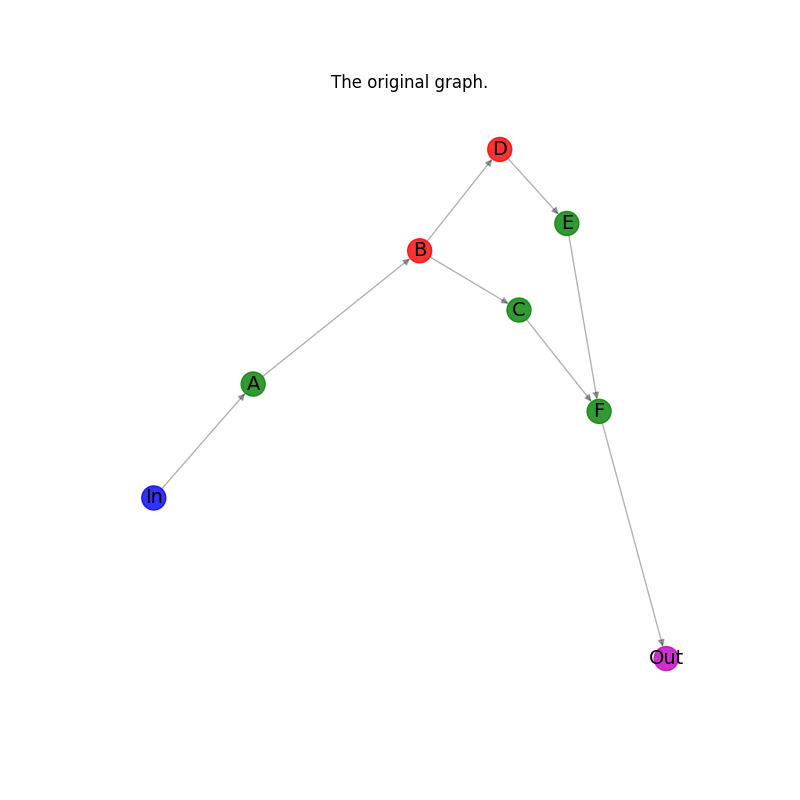## Calculate the subgraphs with plotting all results of intemediate steps.#

```subgraphs_of_G_ex, removed_edges = graph_partitioning(G_ex, plotting=True)
```
•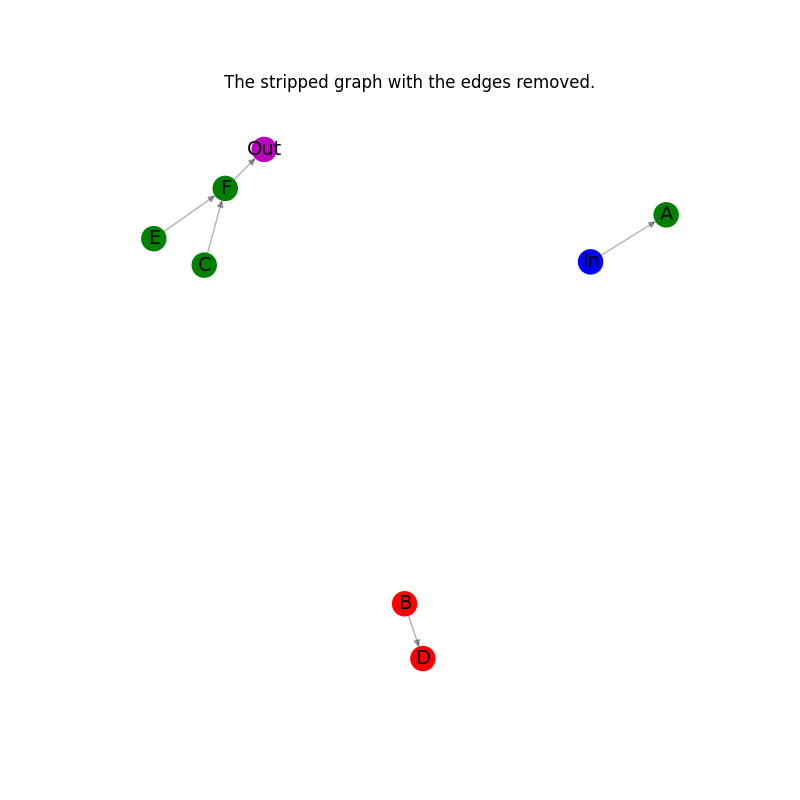•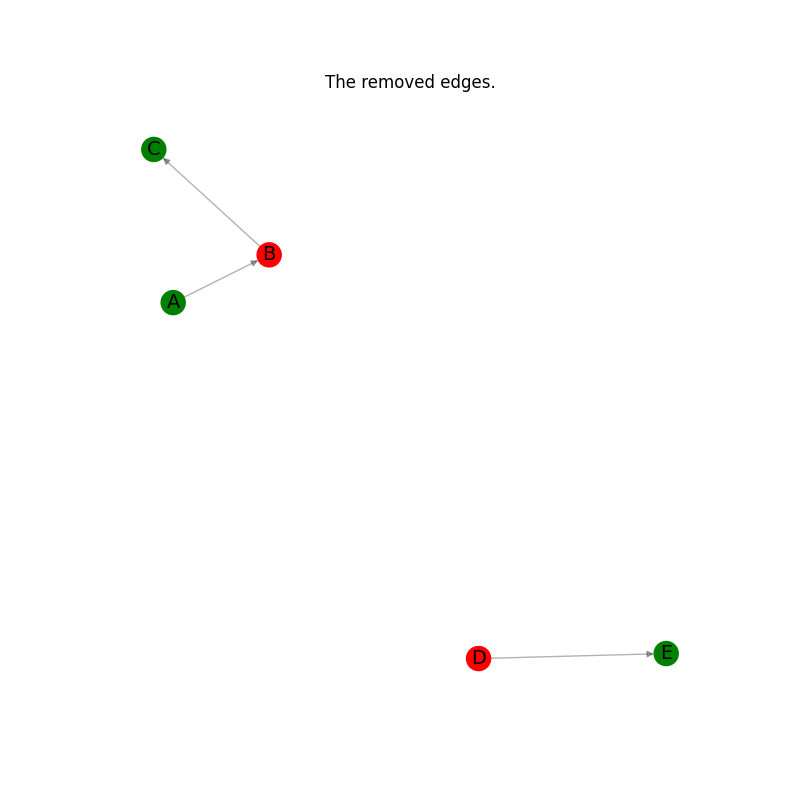## Plot the results: every subgraph in the list.#

```for subgraph in subgraphs_of_G_ex:
_pos = nx.spring_layout(subgraph)
plt.figure(figsize=(8, 8))
nx.draw_networkx_edges(subgraph, _pos, alpha=0.3, edge_color="k")
node_color_list_c = [nc for _, nc in subgraph.nodes(data="node_color")]
nx.draw_networkx_nodes(subgraph, _pos, node_color=node_color_list_c)
nx.draw_networkx_labels(subgraph, _pos, font_size=14)
plt.axis("off")
plt.title("One of the subgraphs.")
plt.show()
```
•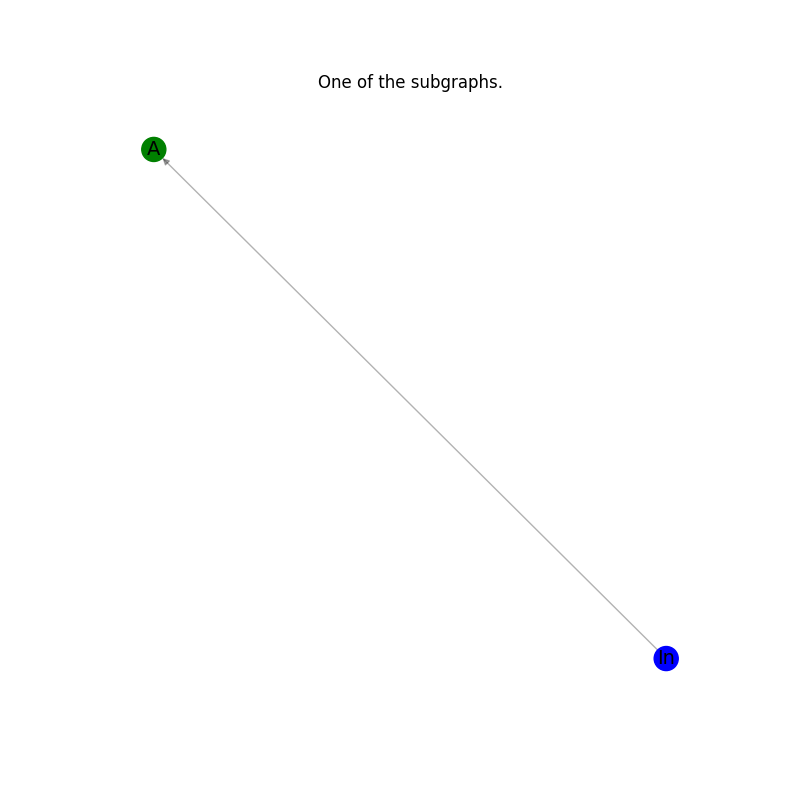•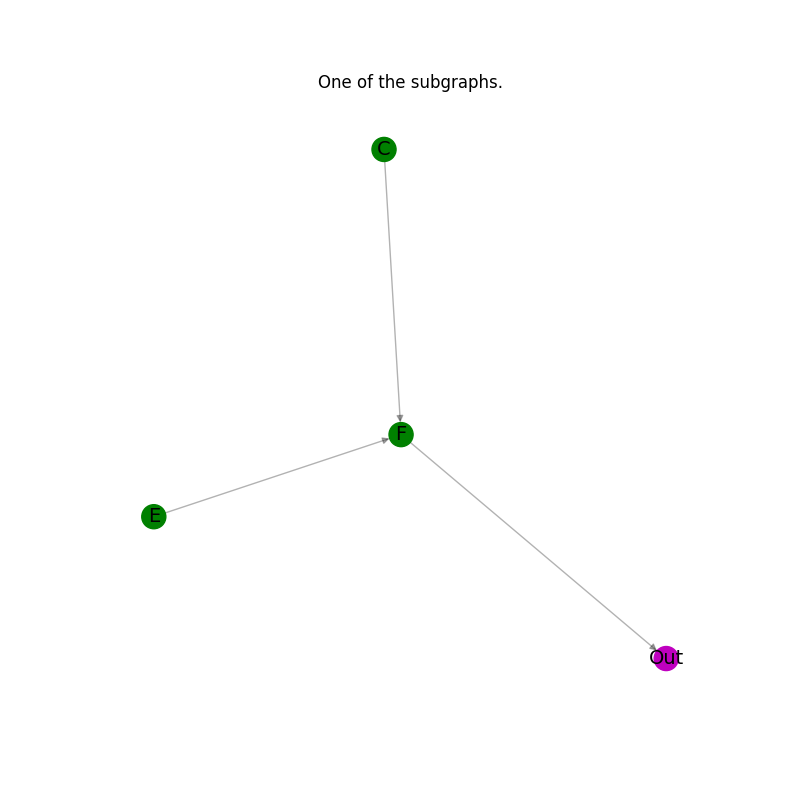•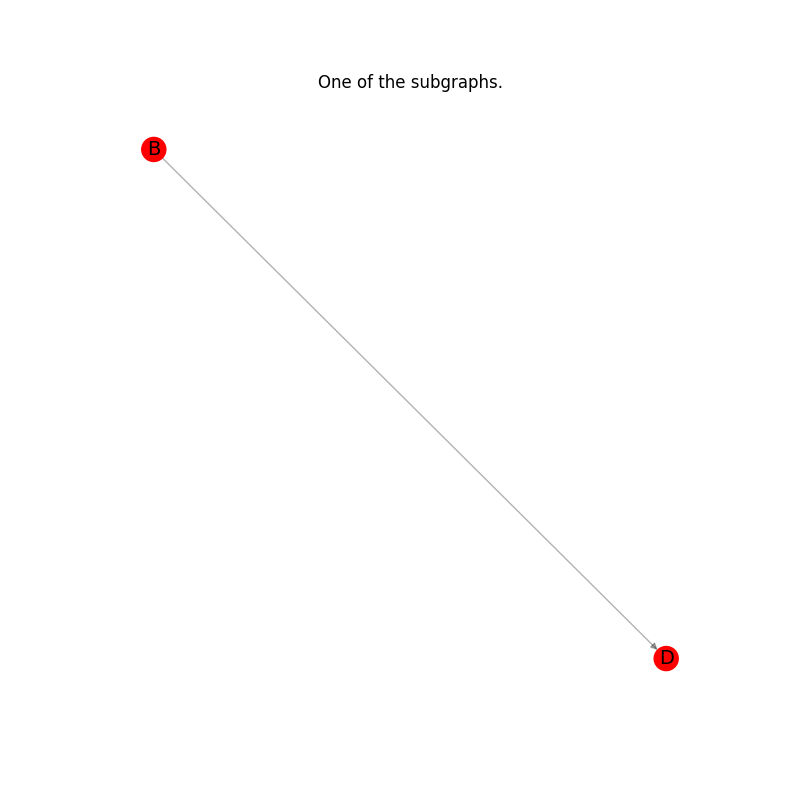## Put the graph back from the list of subgraphs#

```G_ex_r = nx.DiGraph()
# Composing all subgraphs.
for subgraph in subgraphs_of_G_ex:
G_ex_r = nx.compose(G_ex_r, subgraph)
# Adding the previously stored edges.
```

## Check that the original graph and the reconstructed graphs are isomorphic.#

```assert nx.is_isomorphic(G_ex, G_ex_r)
```

## Plot the reconstructed graph.#

```node_color_list = [nc for _, nc in G_ex_r.nodes(data="node_color")]
pos = nx.spectral_layout(G_ex_r)
plt.figure(figsize=(8, 8))
nx.draw_networkx_edges(G_ex_r, pos, alpha=0.3, edge_color="k")
nx.draw_networkx_nodes(G_ex_r, pos, alpha=0.8, node_color=node_color_list)
nx.draw_networkx_labels(G_ex_r, pos, font_size=14)
plt.axis("off")
plt.title("The reconstructed graph.")
plt.show()
```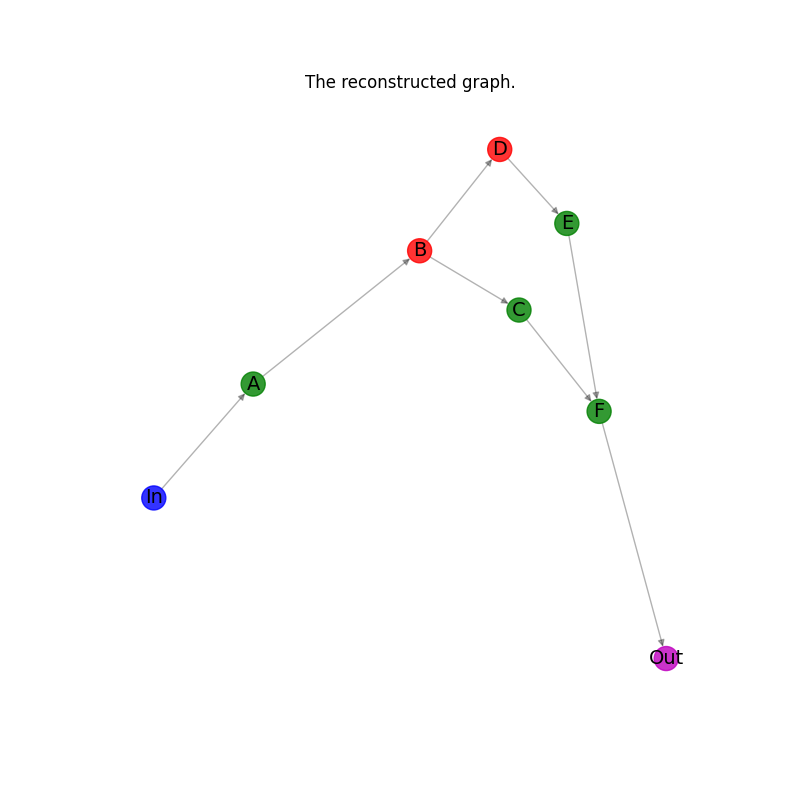Total running time of the script: ( 0 minutes 0.716 seconds)

Gallery generated by Sphinx-Gallery